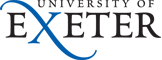Physics and Astronomy
Home Our Teaching Resources CDHW Feedback PracticalMatters.html

## Practical Matters

Previous: Types of Feedback Control.

In its raw form integral control can be a mixed blessing; if the error TsTo is large for a long period, for example after a large change in Ts or at switch-on, the value of the integral can become excessively large and cause overshoot or undershoot that takes a long time to recover. To avoid this problem, which is often called 'integral wind-up', sophisticated controllers will inhibit integral action until the system gets fairly close to equilibrium. One method of achieving this is used by the interactive simulation: when the "Limit I?" option is selected the value of the integral is held constant during periods when the heater is at maximum, or zero, power. This technique seems quite effective and would be straightforward to incorporate in a real controller.

Any system using a resistive electrical heater to control temperature is inherently non-linear because an electrical heater can only generate, not absorb, heat. When the oven temperature is higher than the set-point cooling occurs at a rate that depends on the oven and its temperature not the controller and dual PID controllers allow different heating and cooling parameter values to cope with this. It is possible to build your own PID controller from a few operational-amplifiers. Commercial PID process controllers vary in cost between £75 for a simple model and £600 for an intelligent autotuning dual PID model.

Don't just assume that the knobs on a PID controller correspond to the parameters defined in this document. Values are sometimes specified by time constants in which case a long integral time constant is equivalent to a low value of I but a long derivative time constant means a large value of D. The proportional gain is sometimes set by choosing a proportional band which is the change in input that gives maximum change in heater power so a small number for this corresponds to a large value of P.

### Varieties of PID Algorithms

The parallel algorithm variety of PID control, the version discussed in this doument

$$W = P \times \left ( \left ( T_{\rm s} - T_{\rm o} \right) + D \times {{\rm d}\over {\rm dt}} \left ( T_{\rm s} - T_{\rm o} \right) + I \times \int{ \left ( T_{\rm s} - T_{\rm o} \right)\,\rm d t} \right )$$

is often referred to as the 'ideal algorithm'. To implement this scheme accurately one needs at least three amplifiers (the example controller circuit uses five). However, if slight deviations from the 'ideal' behaviour are permitted, only one amplifier is needed. This can be a great advantage, particularly in pneumatic systems where amplifiers are expense items. Differences in the achievable control performance due to which algorithm is being used are not normally significant. However, the tuning procedures used do differ slightly. Also, some controllers only apply derivative action to the process variable, not to the set point. Whether this is an advantage or not depends on the circumstances. For more details, refer to David St Clair's comparison of different implementations of the PID algorithm.

### Control Theory

Avoid re-inventing the wheel when tackling difficult feedback or control problems - control theory is a well-developed branch of engineering and has a range of powerful techniques to design and analyse systems involving feedback. As well as having systematic methods for solving complicated problems it introduces the important ideas of controllability ('Is it possible to control X by adjusting Y?'), observability ('Does the system have distinct states that can't be unambiguously identified by the controller?') and robustness ('Will control be regained satisfactorily after an unexpected disturbance?').

### Noise and the Frequency Domain

The frequency domain behaviour of the model can be investigated with the interactive simulator which will plot the open- and closed-loop frequency response for the system. As the controller gain is increased the phase margin reduces towards zero causing the overshoot described previously and a resonant peak in the frequency domain response. Any additional lag in the system, for example a non-negligible time-constant for the sensor measuring To, will make it possible for the system to oscillate, which is the reason for the second step in the procedure suggested for tuning a PID controller. Note that integral action reduces the phase margin, derivative action improves it. Even if a system is technically stable it is unwise to operate it with a large peak in the closed-loop gain as this will act as an amplifier for any sensor noise and may cause large and undesired fluctuations in the heater power. If you have a noisy system to control you almost certainly do not want to use any derivative action.